# Example file of how to use adaptive sparse grid for HJB Equation

by SeHyoun Ahn, July 2016

## Contents

% Requires: <generate_spgrid.m>
%
%           <gen_diff_op.m>
%
%           <grid_evaluation.m>
%


## Set up Grids

n_dim = 2;                 % Dimensionality of the problem
n_levels = 4;              % Level of the starting sparse grid
I = 100;                   % Number of asset grid points for full grid
J = 40;                    % Number of skill grid points for full grid

% Generate sparse grid
[spgrid,spspace] = generate_spgrid(n_dim,n_levels);

% Setup Change of basis from Hierachical to Nodal
hier_to_nodal = grid_evaluation(spgrid,spgrid,spspace);

% Generate Difference Operators
[forward_diff_x,backward_diff_x] = gen_diff_op(1,spgrid,spspace,min(spspace(:,1)));
[forward_diff_y,backward_diff_y] = gen_diff_op(2,spgrid,spspace,min(spspace(:,2)));

% Second Derivative Operator
% More tests are needed to check which stencil is the best for second order
% Operators
second_deriv_y = forward_diff_y*(hier_to_nodal\backward_diff_y) ...
+ backward_diff_y*(hier_to_nodal\forward_diff_y);
second_deriv_y(spgrid(:,2)==1,:) = -backward_diff_y(spgrid(:,2)==1,:);
second_deriv_y(spgrid(:,2)==0,:) = forward_diff_y(spgrid(:,2)==0,:);

% Integration Weights
% This does not get used here
int_weight = (prod(spspace,2)./2.^sum(spspace==1,2))';


## Set up Model

Parameters

ga = 2;             % CRRA utility with parameter gamma
alpha = 0.35;       % Production function F = K^alpha * L^(1-alpha)
delta = 0.1;        % Capital depreciation
zmean = 1.0;        % mean O-U process (in levels). This parameter has to be adjusted to ensure that the mean of z (truncated gaussian) is 1.
sig2 = (0.10)^2;    % sigma^2 O-U
Corr = exp(-0.3);   % persistence -log(Corr)  O-U
rho = 0.05;         % discount rate
TFP = 1;

K = 3.8;            % initial aggregate capital. It is important to guess a value close to the solution for the algorithm to converge

zmin = 0.5;   % Range z
zmax = 1.5;
amin = -1;    % borrowing constraint
amax = 30;    % range a

%simulation parameters
maxit  = 100;       %maximum number of iterations in the HJB loop
maxitK = 100;       %maximum number of iterations in the K loop
crit = 10^(-10);    %criterion HJB loop
critK = 1e-7;       %criterion K loop
Delta = 1000;       %delta in HJB algorithm

% Ornstein-Uhlenbeck
the = -log(Corr);
Var = sig2/(2*the);

% Evalute asset and skill values for grid points
aa = spgrid(:,1)*(amax-amin)+amin;
zz = spgrid(:,2)*(zmax-zmin)+zmin;
mu = the*(zmean-zz);
s2 = sig2;

% Differential operator for skill
Aswitch = bsxfun(@times,mu.*(mu>=0),forward_diff_y)/(zmax-zmin) ...
+ bsxfun(@times,mu.*(mu<0),backward_diff_y)/(zmax-zmin) ...
+ s2/2*second_deriv_y/(zmax-zmin)^2;

% Initial guess
r = alpha     * TFP * K^(alpha-1) -delta; %interest rates
w = (1-alpha) * TFP * K^(alpha);          %wages
v0 = (w.*zz + r.*aa).^(1-ga)/(1-ga)/rho;
v = hier_to_nodal\v0;
dist = zeros(1,maxit);
V=v;

number_of_points=0;


## Iterate to Solve HJB Equation Adding Nodes to Sparse Grids as Needed

Note that the Iteration Part of the Code is the same except for difference operator

while true

  for n=1:maxit
Vaf = forward_diff_x*V/(amax-amin);
Vab = backward_diff_x*V/(amax-amin);

c0 = w*zz + r.*aa;
cf = Vaf.^(-1/ga);
sf = w*zz + r.*aa - cf;
cb = Vab.^(-1/ga);
sb = w*zz + r.*aa - cb;

If = (sf >  0).*(spgrid(:,1)<1);
Ib = (sb < 0).*(If==0).*(spgrid(:,1)>0);
I0 = (1-If-Ib);

c = cf.*If + cb.*Ib + c0.*I0;
u = c.^(1-ga)/(1-ga);

AA = bsxfun(@times,If.*sf,forward_diff_x)/(amax-amin) + bsxfun(@times,Ib.*sb,backward_diff_x)/(amax-amin);

B = (1/Delta + rho)*hier_to_nodal - AA - Aswitch;

b = u + hier_to_nodal*V/Delta;

V_new = B\b;

Vchange = V_new - V;
V = V_new;

dist = max(max(abs(Vchange)));
if dist<crit
disp('Value Function Converged, Iteration = ')
disp(n)
break
end
end

% Plot Value function given the grid
figure(1);
scatter3(aa,zz,hier_to_nodal*V);
drawnow;

V_nodal = hier_to_nodal*V;

Value Function Converged, Iteration =
9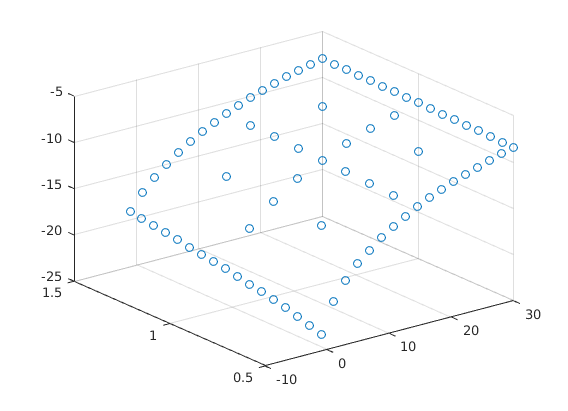Add new nodes to sparse grid if needed

  % Find Position to Expand
loc = abs(V)>1e-4*(max(V_nodal)-min(V_nodal));

% Compute New Grid Points from active grid points
[new_points,new_spaces] = gen_new_points(spgrid(loc,:),spspace(loc,:));

% Find Positions to Compress Away
loc_keep = abs(V)>8e-5*(max(V_nodal)-min(V_nodal));
[xx,loc] = unique([spgrid(loc_keep,:);new_points],'rows');

% Break if no new grid points are added
if number_of_points >= size(xx,1)
break;
end
number_of_points = size(xx,1);

% Update interpolation operator
x_eval = grid_evaluation(xx,spgrid,spspace);
v_nodal = x_eval*V;
spgrid = xx;
spspace = [spspace(loc_keep,:);new_spaces];
spspace = spspace(loc,:);
hier_to_nodal = grid_evaluation(xx,spgrid,spspace);

% Correct the basis function for the new bases
V = hier_to_nodal\v_nodal;

% Update Difference Operators for the new grid
[forward_diff_x,backward_diff_x] = gen_diff_op(1,spgrid,spspace,min(spspace(:,1)));
[forward_diff_y,backward_diff_y] = gen_diff_op(2,spgrid,spspace,min(spspace(:,2)));
second_deriv_y = forward_diff_y*(hier_to_nodal\backward_diff_y) ...
+ backward_diff_y*(hier_to_nodal\forward_diff_y);
second_deriv_y(spgrid(:,2)==1,:) = -backward_diff_y(spgrid(:,2)==1,:);
second_deriv_y(spgrid(:,2)==0,:) = forward_diff_y(spgrid(:,2)==0,:);

% Update Nodal Values for new grid
aa = spgrid(:,1)*(amax-amin)+amin;
zz = spgrid(:,2)*(zmax-zmin)+zmin;
mu = the*(zmean-zz);
s2 = sig2;

Aswitch = bsxfun(@times,mu.*(mu>=0),forward_diff_y)/(zmax-zmin) ...
+ bsxfun(@times,mu.*(mu<0),backward_diff_y)/(zmax-zmin) ...
+ s2/2*second_deriv_y/(zmax-zmin)^2;

end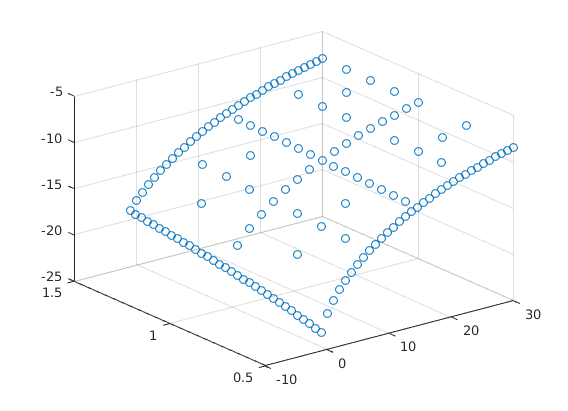Value Function Converged, Iteration =
7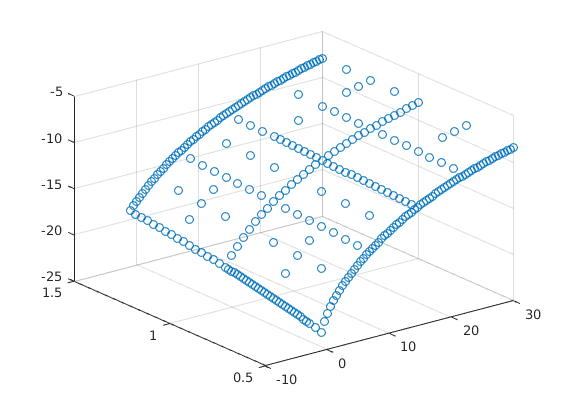Value Function Converged, Iteration =
8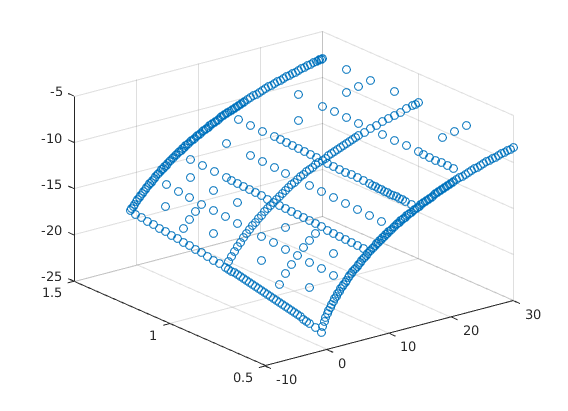Value Function Converged, Iteration =
8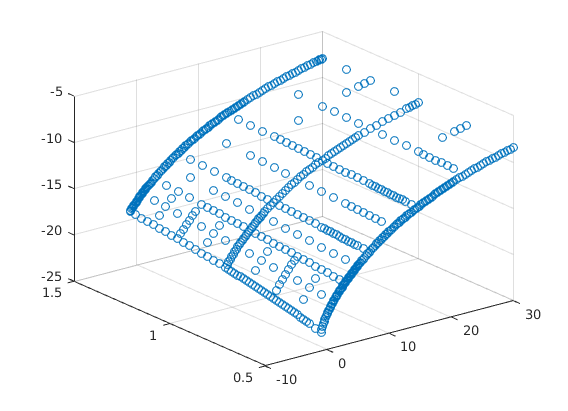Value Function Converged, Iteration =
9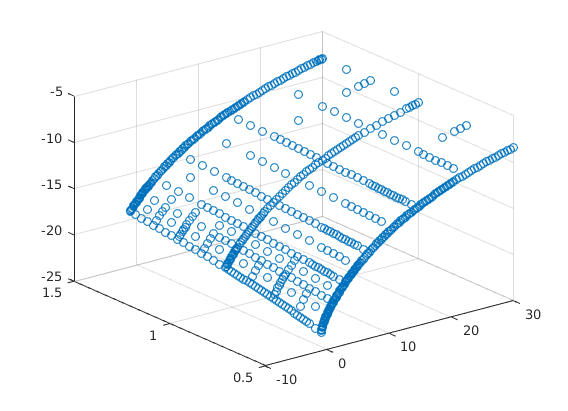Value Function Converged, Iteration =
8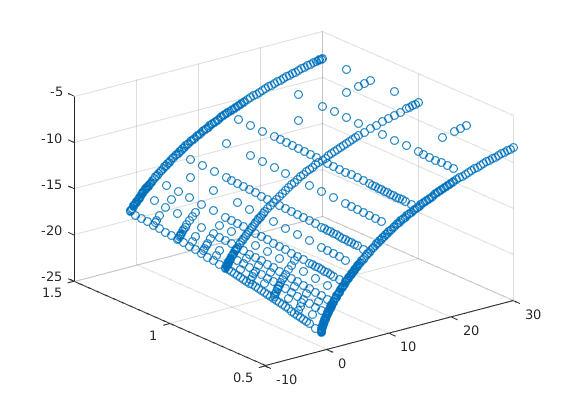Value Function Converged, Iteration =
8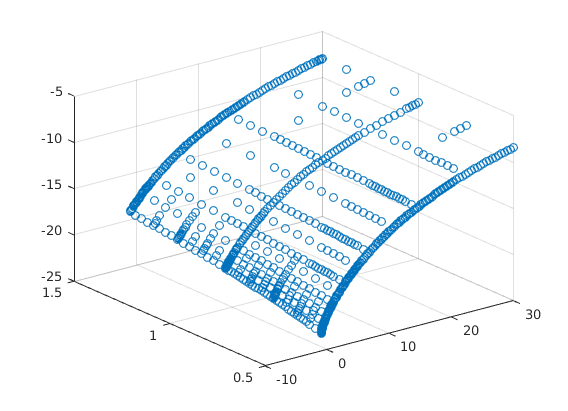Value Function Converged, Iteration =
7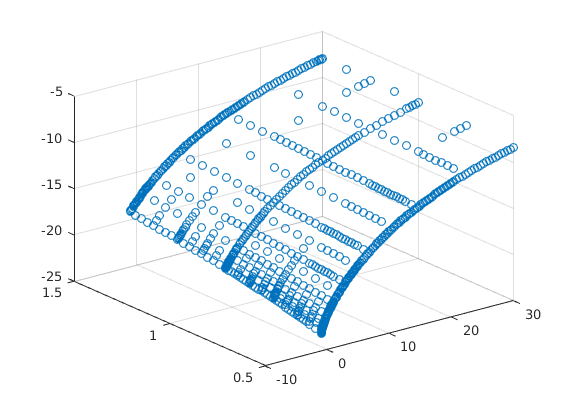Value Function Converged, Iteration =
6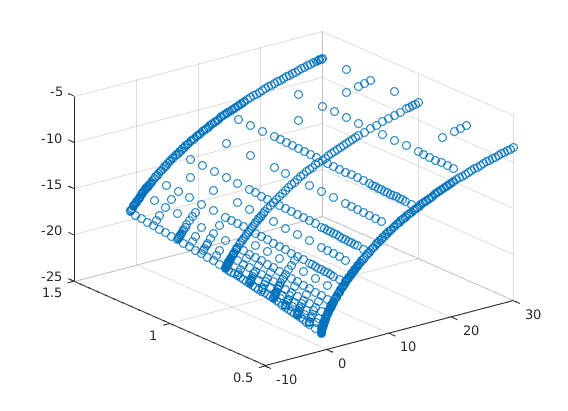Value Function Converged, Iteration =
7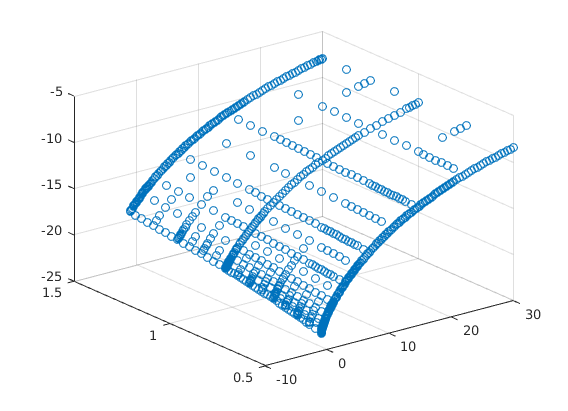figure(2);
scatter3(aa,zz,hier_to_nodal*V);
V_sparse = V;Comparison Plot to Full GridThe following code is adapted from Ben Moll's codes available at     https://www.princeton.edu/~moll/HACTproject.htma = linspace(amin,amax,I)';    % wealth vector
da = (amax-amin)/(I-1);
z = linspace(zmin,zmax,J);     % productivity vector
dz = (zmax-zmin)/(J-1);
dz2 = dz^2;
aa = a*ones(1,J);
zz = ones(I,1)*z;

mu = the*(zmean - z);        % Drift
s2 = sig2.*ones(1,J);        % Variance

% Preallocation
Vaf = zeros(I,J);
Vab = zeros(I,J);
Vzf = zeros(I,J);
Vzb = zeros(I,J);
Vzz = zeros(I,J);
c = zeros(I,J);

yy = - s2/dz2 - mu/dz;
chi =  s2/(2*dz2);
zeta = mu/dz + s2/(2*dz2);

updiag=zeros(I*J,1); %This is necessary because of the peculiar way spdiags is defined.
updiag(I+1:I*J)=reshape(repmat(zeta(1:J-1),I,1),I*(J-1),1);

centdiag=zeros(I*J,1);
centdiag(1:I)=repmat(chi(1)+yy(1),I,1);
centdiag(I+1:I*(J-1))=reshape(repmat(yy(2:J-1),I,1),I*(J-2),1);
centdiag(I*J-I+1:I*J)=repmat(yy(J)+zeta(J),I,1);

lowdiag=reshape(repmat(chi(2:J),I,1),I*(J-1),1);

Aswitch=spdiags(centdiag,0,I*J,I*J)+spdiags(lowdiag,-I,I*J,I*J)+spdiags(updiag,I,I*J,I*J);

% Initial Guess
r = alpha     * TFP * K^(alpha-1) -delta; %interest rates
w = (1-alpha) * TFP * K^(alpha);          %wages
v0 = (w*zz + r.*aa).^(1-ga)/(1-ga)/rho;
v = v0;

for n=1:maxit
V = v;

% forward difference
Vaf(1:I-1,:) = (V(2:I,:)-V(1:I-1,:))/da;
Vaf(I,:) = (w*z + r.*amax).^(-ga); %will never be used, but impose state constraint a<=amax just in case

% backward difference
Vab(2:I,:) = (V(2:I,:)-V(1:I-1,:))/da;
Vab(1,:) = (w*z + r.*amin).^(-ga);  %state constraint boundary condition

%consumption and savings with forward difference
cf = Vaf.^(-1/ga);
sf = w*zz + r.*aa - cf;
%consumption and savings with backward difference
cb = Vab.^(-1/ga);
sb = w*zz + r.*aa - cb;
%consumption and derivative of value function at steady state
c0 = w*zz + r.*aa;
Va0 = c0.^(-ga);

If = sf > 0; %positive drift --> forward difference
Ib = sb < 0; %negative drift --> backward difference
I0 = (1-If-Ib); %at steady state

Va_Upwind = Vaf.*If + Vab.*Ib + Va0.*I0; %important to include third term

c = Va_Upwind.^(-1/ga);
u = c.^(1-ga)/(1-ga);

X = - min(sb,0)/da;
Y = - max(sf,0)/da + min(sb,0)/da;
Z = max(sf,0)/da;

Z(I,:)=0;
X(1,:)=0;
updiag=[0;reshape(Z,I*J,1)];
centdiag=reshape(Y,I*J,1);
lowdiag=reshape(X,I*J,1);

AA=spdiags(centdiag,0,I*J,I*J)+spdiags([updiag;0],1,I*J,I*J)+spdiags(lowdiag(2:I*J),-1,I*J,I*J);
A = AA + Aswitch;
B = (1/Delta + rho)*speye(I*J) - A;

u_stacked = reshape(u,I*J,1);
V_stacked = reshape(V,I*J,1);

b = u_stacked + V_stacked/Delta;

V_stacked = B\b;

V = reshape(V_stacked,I,J);

Vchange = V - v;
v = V;

dist = max(max(abs(Vchange)));
if dist<crit
disp('Value Function Converged with Full Grid, Iteration = ');
disp(n);
break;
end
end

h = figure(2);
hold on;
surf(a,z,V');
title('Comparison to Uniform Grid');
gif_maker_rotate('comparison.gif',h);
Value Function Converged with Full Grid, Iteration =
8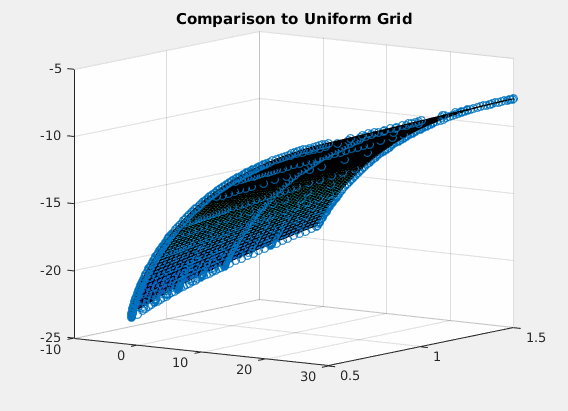Published with MATLAB® R2016a (and adjusted not to clash with Bootstrap)